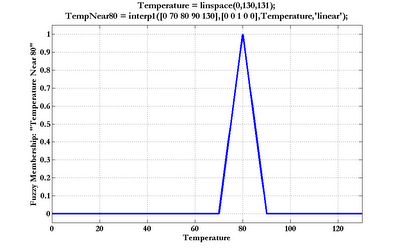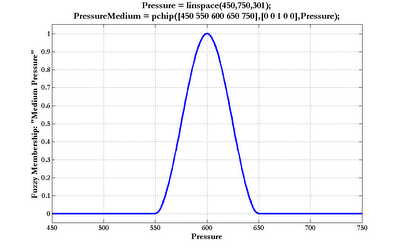## Thursday, November 16, 2006

### Fuzzy Logic In MATLAB Part 1

Fuzzy logic is a capable and often misunderstood analytical tool. A basic grasp of this technology may be gained from any of the following introductions to fuzzy logic:

Putting Fuzzy Logic to Work (PDF), PC AI magazine, by Dwinnell

Fuzzy Math, Part 1, The Theory, by Luka Crnkovic-Dodig

Fuzzy Logic Introduction (PDF), by Martin Hellmann

Fuzzy Logic Overview (HTML)

comp.ai.fuzzy FAQ: Fuzzy Logic and Fuzzy Expert Systems

Fuzzy Logic for "Just Plain Folks" (HTML), by Thomas Sowell

As a rule, in the interest of portability and transparency, I try to build as much of my code as possible in base MATLAB, and resort to using toolboxes and such only when neccessary. Fortunately, fuzzy logic is exceedingly easy to implement in the base MATLAB product.

Generation of fuzzy set memberships can be accomplished using the base MATLAB functions interp1 and pchip. Piecewise linear (most commonly: triangular and trapezoidal) fuzzy memberships can be calculated using the 'linear' method in interp1. Below is an example of a triangular fuzzy memebership function, defined over the Temperature domain (click the graph to enlarge):The domain variable and shape parameters (domain vector followed by membership vector) control the form of the curve. Trapezoids are constructed by adding another element to each shape vector, like this:

Temperature = linspace(0,130,131);
Temp80ish = interp1([0 70 78 82 90 130],[0 0 1 1 0 0],Temperature,'linear');

Fuzzy membership functions with smoother transitions are possible by using the pchip function. The following depicts a bell-shaped membership function (as usual, click the graph to enlarge):Of course, one is not limited to peaked or plateau-shaped fuzzy membership functions. The various interpolation and spline functions in MATLAB permit whatever irregular and arbitrary shapes may be called for.

In Part 2, I will build a small fuzzy rule base in MATLAB.

Print:

An excellent practical reference:
The Fuzzy Systems Handbook (Second Edition), by Cox (ISBN: 0121944557)Anonymous said...

great blog ... and thank in advance
it's perfect and i have'nt any idea for improve just more focus on application and implementing this issue (fuzzy/ga ...),anyway thank again

Earl Cox said...

Will, thanks for the plug, but Rod and Mike never stepped up to the the project so, alas, they were not co-authors of my second edition. Just me.

And should I send you a copy of the new book, Fuzzy Models and Genetic Algorithms for Data Mining and Exploration?

Will Dwinnell said...

Duly noted and corrected, thanks!

Pankhi said...

hi,
I am doing a project on CBIR using Fuzzy Logic in Matlab. I am new to this field and have some queries regarding using Matlab. I would really appreciate if i can get some help from you. Please contact me if you can. My email id is pankhi.2009@gmail.com. Waiting eagerly for your response.